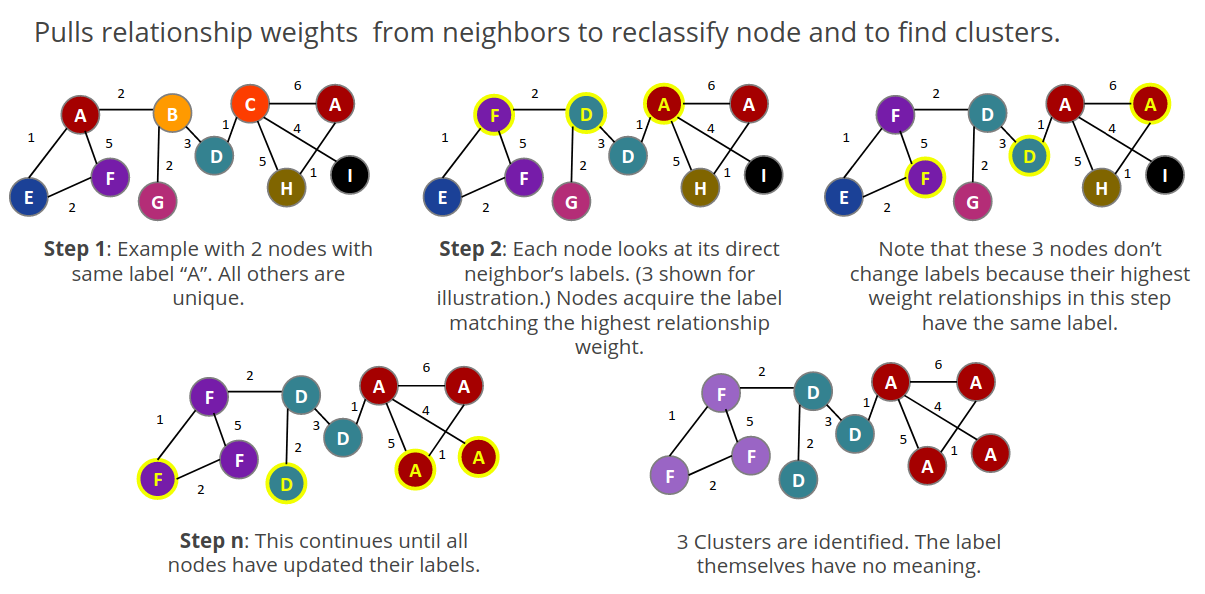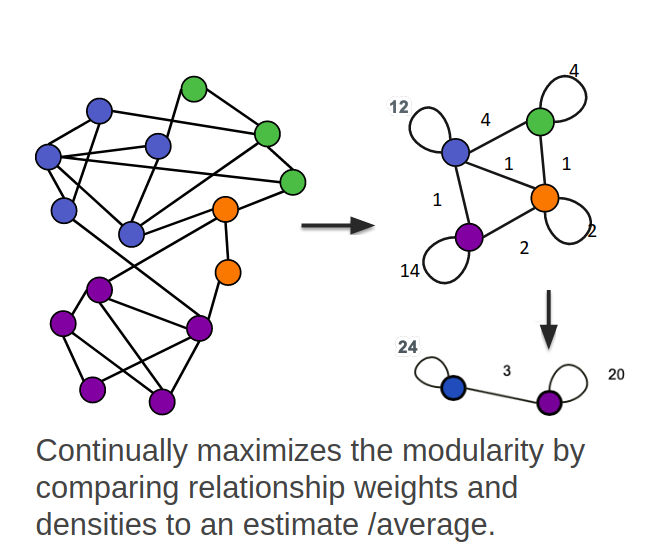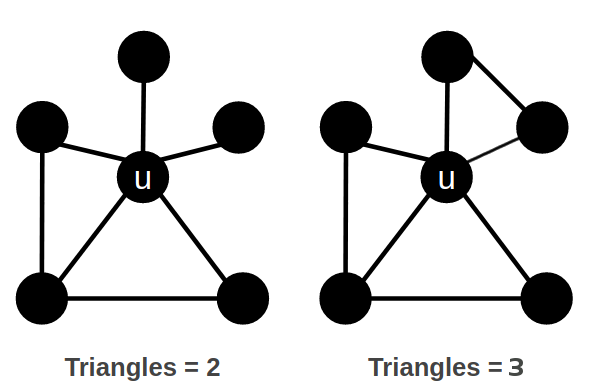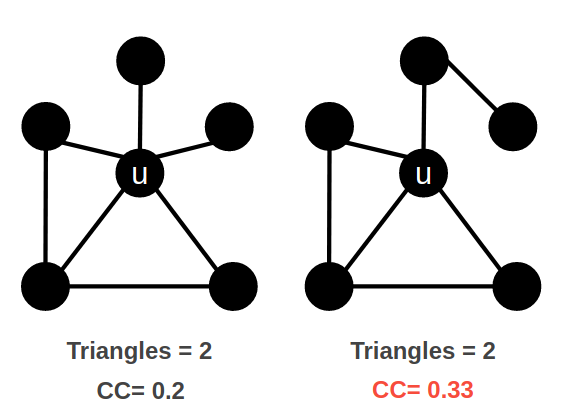# Community Detection Algorithms

## Community Detection algorithms

Community Detection algorithms are designed to help you discover and understand the structure of complex networks.

Understanding the community structure has many real-world applications in sociology, biology, and computer science. You can think of a community as a densely-connected group of nodes, similar to how a group of friends is highly interconnected.

These algorithms are supported:

• Weakly Connected Components (unionFind)

• Label Propagation

• Louvain Modularity

• Triangle Counting

• Local Clustering Coefficient

Labs implementations:

• Strongly Connected Components

• K-1 Coloring

• Modularity optimization

## Weakly Connected Components

The Weakly Connected Components (WCC) algorithm is used to find disconnected subgraphs or islands within our network.

In a single connected component, each node can reach all other nodes when disregarding relationships' direction, effectively treating the connections as undirected. Understanding how many connected components exist in our network is crucial to understanding the graph structure. For that reason, the WCC algorithm is often used early in graph analysis. A best practice is to run WCC to test whether a graph is connected as a preparatory step for all other graph algorithms. Performing this quick test can avoid accidentally running algorithms on only one disconnected component of a graph and getting incorrect results. It can also help you to better comprehend results from other algorithms.

### Why use Weakly Connected Components?

Here is why you use WCC:

• Early step in graph analysis to see how a network is connected

• Fraud detection

Here are some examples:

• Finding common identifiers in disambiguation process.

• Identifying disconnected subgraphs to run resource intensive algorithms (like Node Similarity) in parallel.

## Guided Exercise: Getting Started with the Weakly Connected Component algorithm

Follow along with this video to run the Connected Components algorithm using the Graph Data Science Playground (NEuler).

 Before you perform the tasks in this Guided Exercise, you must have either created and started the database in the Neo4j Desktop, and followed the instructions for loading the data in the Browser Guide: `:play 4.0-intro-graph-algos-exercises`.

### Exercise: Weakly Connected Components

1. In NEuler:

1. Find all Connected Characters for Season 3 writing the unionFind_season3 property in the Game of Thrones data.

2. Edit the configuration and find all Connected Character nodes, but leave the Any relationship type, writing the unionFind_any value.

2. In Neo4j Browser: :play 4.0-intro-graph-algos-exercises and follow the instructions for Weakly Connected Components.

 Estimated time to complete: 15 minutes

## Label Propagation

The Label Propagation algorithm (LPA) is a fast algorithm for describing communities in a graph. It detects these groups using network topology alone, and does not require any prior information about the communities, or their structure.

In the LPA, the nodes select their community based on their direct neighbors using the node labels. In addition, weights on nodes and relationships can also be considered. The idea is that a single label can quickly become dominant in a densely-connected group of nodes, but it will have trouble crossing a sparsely connected region.Here is how the Label Propagation algorithm works. First, every node is initialized with a property. By default, the initial property is unique for every node. However, the LPA also lends itself well to semi-supervised learning because you can seed the initial properties with pre-assigned node labels that you know are predictive.

In this example, we have started with 2 A nodes, but left all other nodes as unique. We are using the node default weights of 1. Nodes are then processed randomly, with each node acquiring its neighbor’s label with the maximum weight. So, in the first iteration, the left A acquires the label F, B acquires the label D, and C now becomes A. The maximum weight is calculated based on the weights of neighbor nodes and their relationships. In addition, ties are broken uniformly and randomly. There will be times when a label is not updated because the neighbor with the maximum weight has the same label. Iterations continue until each node has the majority label of its neighbors or reached the maximum iteration limit. A maximum iteration limit will prevent endless cycles where the algorithm cannot converge on a solution, essentially getting caught in a flip-flop cycle for some labels. In contrast to other algorithms, LPA can return different community structures when run multiple times on the same graph. The order in which LPA evaluates nodes can influence the final communities it returns. Another factor is the random tie-breaking process.

### Why use Label Propagation?

Here is why you use LPA:

• Community detection

• Semi-supervised community detection

• Preprocessing data (classification)

Here are some examples:

• Assigning polarity of tweets as a part of semantic analysis. In this scenario, positive and negative seed labels from a classifier are used in combination with the Twitter follower graph. For more information, see Twitter polarity classification with label propagation over lexical links and the follower graph.

• Finding potentially dangerous combinations of possible co-prescribed drugs, based on the chemical similarity and side effect profiles. This study is found here.

• Label Propagation prediction of drug-drug interactions based on clinical side effects.

## Guided Exercise: Getting Started with the Label Propagation algorithm

Follow along with this video to become familiar with running the Label Propagation algorithm using the Graph Data Science Playground (NEuler).

### Exercise: Label Propagation

1. In NEuler:

1. Perform the Label Propagation algorithm on seasons 1 and 2 of Game of Thrones dataset.

2. In Neo4j Browser: :play 4.0-intro-graph-algos-exercises and follow the instructions for Label Propagation.

 Estimated time to complete: 15 minutes

## Louvain Modularity

The Louvain Modularity algorithm is used to detect communities in large networks. You can think of the algorithm doing a "what if" analysis to try out various groupings with the goal of eventually reaching a global modularity optimum.

The Louvain Modularity algorithm consists of repeated application of two steps. The first step is a “greedy” assignment of nodes to communities, favoring local optimizations of modularity. The modularity score quantifies the quality of an assignment of nodes to communities. This process evaluates how much more densely connected the nodes within a community are, compared to how connected they would be in a random network. It starts by calculating the change in modularity if that node joins a community with each of its immediate neighbors. The node then joins the node with the highest modularity change. The process is repeated for each node until the optimal communities are formed. The second step is defining a new coarse-grained network, based on the communities found in the first step. These two steps are repeated until no further modularity-increasing reassignments of communities are possible.In this example, we can see how the Louvain Modularity algorithm works. First, the algorithm assigns nodes to communities by favoring local optimization of modularity. In our case, the algorithm found four groups of nodes, which are indicated by node color. In the second step, the algorithm merges each group of nodes into a single node. The count of links between nodes within the same community and between various communities is now represented as a weighted relationship between the newly-merged nodes. Once the new network is created, the whole process is repeated until a modularity maximum is reached. The Louvain Modularity algorithm is interesting, because you can observe both the final as well as the intermediate communities that are calculated at the end of each level. It is regarded as a hierarchical clustering algorithm because a hierarchy of communities is produced as a result. As a rule of thumb, the communities on lower levels are smaller and more fine-grained than the communities found on higher and final levels.

### Why use Louvain Modularity?

Here is why you use Louvain:

• Community detection in large networks

• Uncover hierarchical structures in data

• Evaluate different grouping thresholds

Here are some examples:

• Extracting topics from online social platforms, like Twitter and YouTube, based on the co-occurence of terms in documents as part of the topic modeling process.

• Finding hierarchical community structures within the brain’s functional network.

• Evaluating criminal networks and holes in the structure.

## Guided Exercise: Getting Started with the Louvain Modularity algorithm

Follow along with this video to become familiar with running the Louvain Modularity algorithm in Neo4j NEuler.

### Exercise: Louvain Modularity

1. In NEuler: Perform the Louvain Modularity algorithm on seasons 2 and 3 of the Game of Thrones dataset.

2. In Neo4j Browser:

1. View the louvain and intermediate louvain values for GOT.

2. :play 4.0-intro-graph-algos-exercises and follow the instructions for Louvain Modularity.

 Estimated time to complete: 15 minutes

## Triangle Count

A triangle contains three nodes where each node has a connection to the other two. In graph theory terminology, a triangle is equivalent to a 3-clique.

The Triangle Count algorithm counts the number of triangles for each node in the graph.

It has gained popularity in social network analysis, where it is used to measure the cohesiveness and stability of networks. It is also one of the indices used in the computation of the local clustering coefficients.

 The Triangle Count algorithm in the GDSL only finds triangles in undirected graphs.In this example, we count the number of triangles passing through node U. In the left example, two triangles pass through node U. The first triangle consists of node U and left-side nodes and the second triangle consists of node U and bottom-side nodes. In the second example, we connect the top right nodes, which produces another triangle.

### Why use Triangle Count?

Here is why you use Triangle Count:

• Estimating stability

• Part of the Local Clustering Coefficient calculation

• Scoring for machine learning

Here is an example:

Identifying features for classifying a given website as spam content. This is described in Efficient Semi-streaming Algorithms for Local Triangle Counting in Massive Graphs.

## Guided Exercise: Getting Started with the Triangle Count algorithm

Follow along with this video to become familiar with running the Triangle Count algorithm in Neo4j NEuler.

### Exercise: Triangle Count

1. In NEuler:

1. Perform some Triangle Count algorithms on seasons 3 and 4 of Game of Thrones.

2. In Neo4j Browser: :play 4.0-intro-graph-algos-exercises and follow the instructions for Triangle Count.

 Estimated time to complete: 10 minutes

## Local Clustering Coefficient

The Local Clustering Coefficient is the probability that neighbors of a particular node are connected to each other.

The goal of the Local Clustering Coefficient algorithm is to measure how tightly a group is clustered compared to how tightly it could be clustered. The algorithm uses Triangle Count in its calculations, which provides a ratio of existing triangles to possible relationships. A maximum value of 1 indicates a clique, where every node is connected to every other node.The Local Clustering Coefficient describes how many of the node’s neighbors are also connected. In the left example, the probability of node U neighbors being connected is 20%. Node U has five neighbors. If all the neighbors were connected to each other, that would be ten relationships between neighbors. Because there are only two relationships between neighbors, the Local Clustering Coefficient is 0.2.

### Why use Local Clustering Coefficient?

Here is why you use Local Clustering Coefficient:

• Estimating network stability

• Finding structural holes

• Scoring for machine learning

Here are some examples:

## Guided Exercise: Getting Started with the Local Clustering Coefficient algorithm

Follow along with this video to become familiar with running the Local Clustering Coefficient algorithm in Neo4j NEuler.

### Exercise: Local Clustering Coefficient

1. In NEuler: Run some Local Clustering Coefficient algorithms on seasons 1 and 2 of Game of Thrones dataset.

2. In Neo4j Browser: :play 4.0-intro-graph-algos-exercises and follow the instructions for Local Clustering Coefficient.

 Estimated time to complete: 10 minutes

### Question 1

What algorithm do you use to calculate the number of triangles all nodes belongs to?

• Triangle Count

• Louvain Modularity

• Weakly Connected Components

• Label Propagation

### Question 2

What algorithm do you use to find disconnected parts of the network?

• Weakly Connected Components

• Louvain Modularity

• Triangle Count

• Label Propagation

### Question 3

What algorithm can be used to examine the hierarchical community structure a graph?

• Triangle Count

• Label Propagation

• Weakly Connected Components

• Louvain Modularity

## Summary

In this module you gained experience running the Neo4j supported algorithms for Community Detection:

• Weakly Connected Components (unionFind)

• Label Propagation

• Louvain Modularity

• Triangle Count

• Local Clustering Coefficient

You can read more about these algorithms and also the alpha (labs) algorithms in the Graph Data Science documentation